# 实验五 shell脚本编程

2.掌握shell脚本语言的基本语法
3.学习简单的shell脚本编程1. 让用户输入一个字符串，如果用户输入的是 hello ,请输入 hello 用户姓名首字母缩写 ，否则输出
请输入hello

#!/bin/bash

SUM=0

for i in {1..100..2}
do
let SUM=$SUM+$i
done
echo "1-100之间的所有奇数和为: $SUM"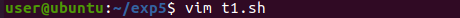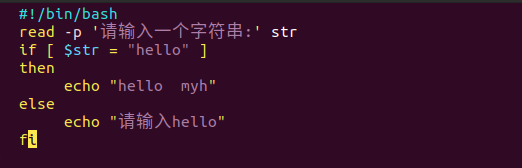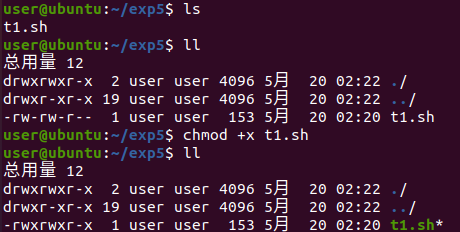2. 编写一脚本，判断输入用户名是否存在，输出结果只能是存在该用户或者不存在该用户，不能有其 他数据输出。 #!/bin/bash read -p '请输入用户名:' user_name id$user_name &> /dev/null
if [ $? -eq 0 ] then echo "存在用户$user_name"
else
echo "不存在用户$user_name" fi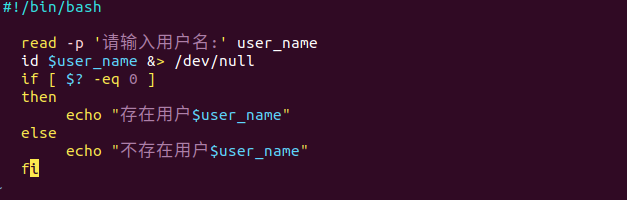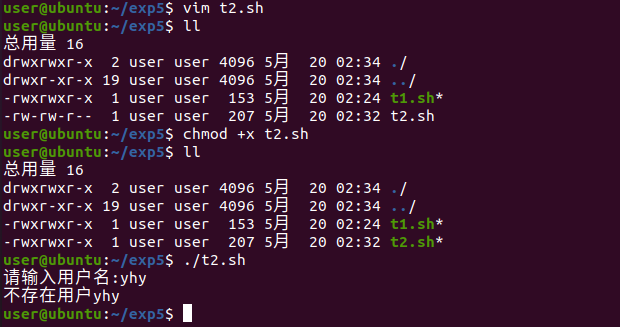3.编写脚本计算1-100之间的所有奇数和 #!/bin/bash SUM=0 for i in {1..100..2} do let SUM=$SUM+$i done echo "1-100之间的所有奇数和为:$SUM"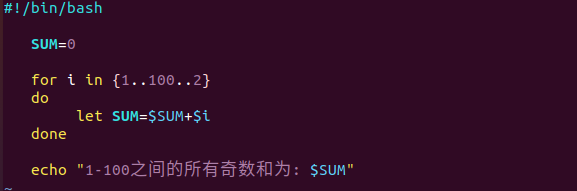4.编写脚本，判断键盘输入整数是否为质数。

#!/bin/bash

[ $num -eq 1 ] && echo '$num是质数'&&exit
[ $num -eq 2 ] && echo '$num是质数'&&exit
for i in seq 2 $[$num-1]
do
 [ $[$num%i] -eq 0 ] && echo "$num不是质数"&&exit done echo "$num是质数"&&exit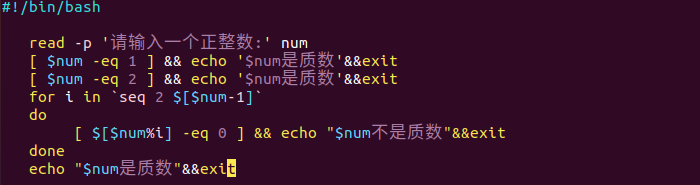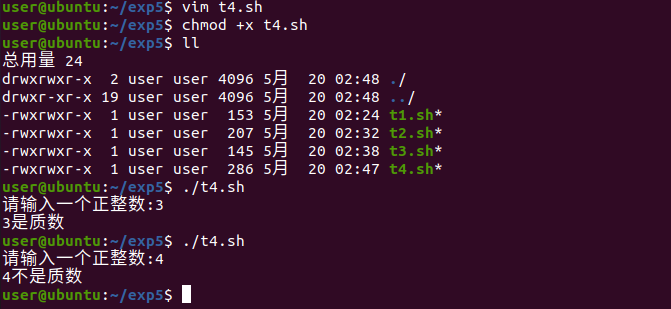5.模拟一个多任务维护界面，当执行程序时先显示总菜单，然后进行选择后做相应的维护监控操作

#!/bin/bash
cat <<-EOF
h 显示命令帮助
f 显示磁盘分区
d 显示磁盘挂载
m 查看内存使用
u 查看系统负载
q 退出程序
EOF
while true
do
case $action in h) cat <<-EOF h 显示命令帮助 f 显示磁盘分区 d 显示磁盘挂载 m 查看内存使用 u 查看系统负载 q 退出程序 EOF ;; f) lsblk ;; d) df -h ;; m) free -m ;; u) uptime ;; q) exit ;; esac done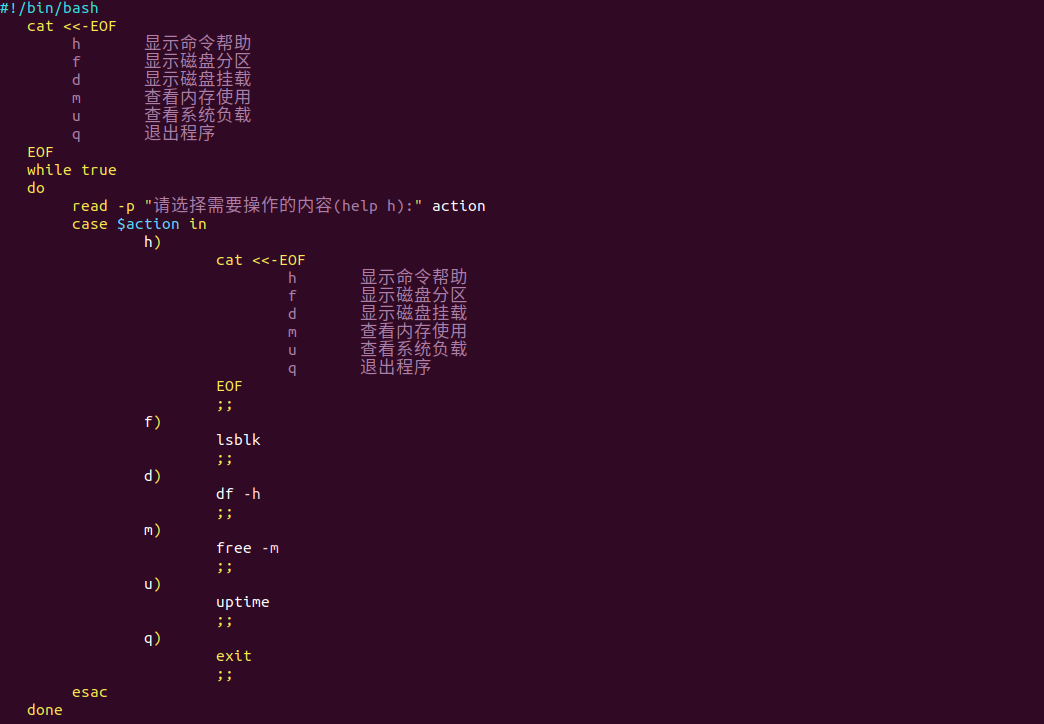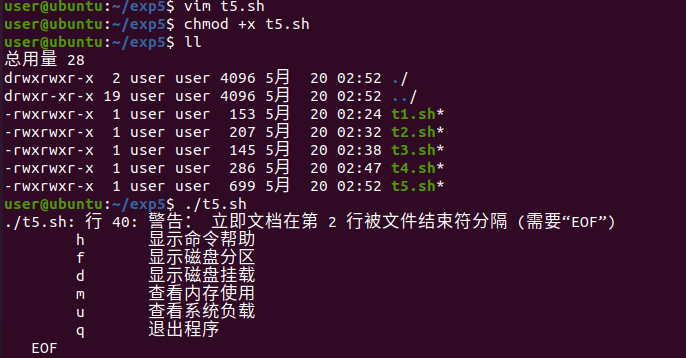6.编写脚本实现计算用户输入参数数字的和，分别用shift和for循环实现。 #!/bin/bash SUM=0 while [$# -ne 0 ]
do
let SUM=$SUM+$1
shift
done
echo SUM=$SUM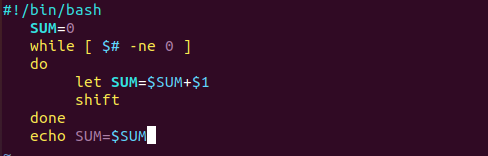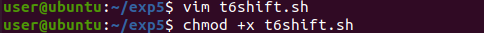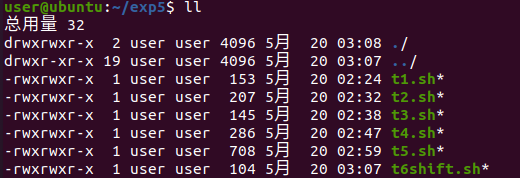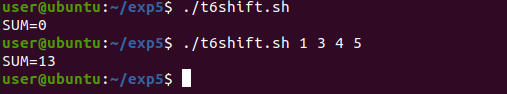#!/bin/bash SUM=0 for i do let SUM=SUM+$i
done
echo SUM=$SUM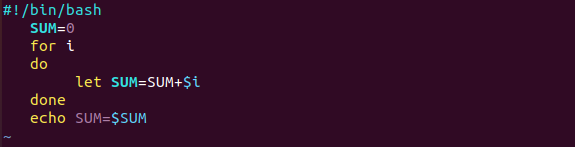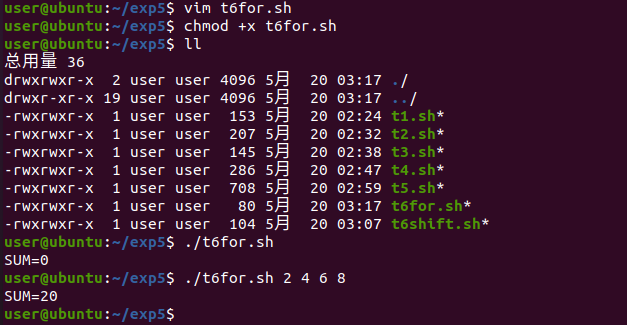7.编写脚本，生成一个phonenumber.txt文件，文件内容为随机产生以139开头的手机号1000个，每个手机号占一行。 #!/bin/bash file=./phonenumber.txt for ((i=1;i<=1000;i++)) do n1=$[ $RANDOM%10] n2=$[ $RANDOM%10] n3=$[ $RANDOM%10] n4=$[ $RANDOM%10] n5=$[ $RANDOM%10] n6=$[ $RANDOM%10] n7=$[ $RANDOM%10] n8=$[ $RANDOM%10] echo "139$n1$n2$n3$n4$n5$n6$n7$n8" >>$file
done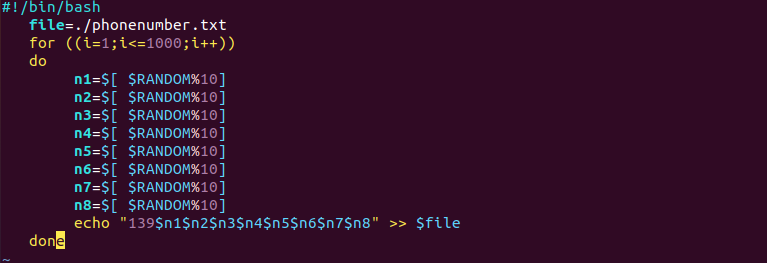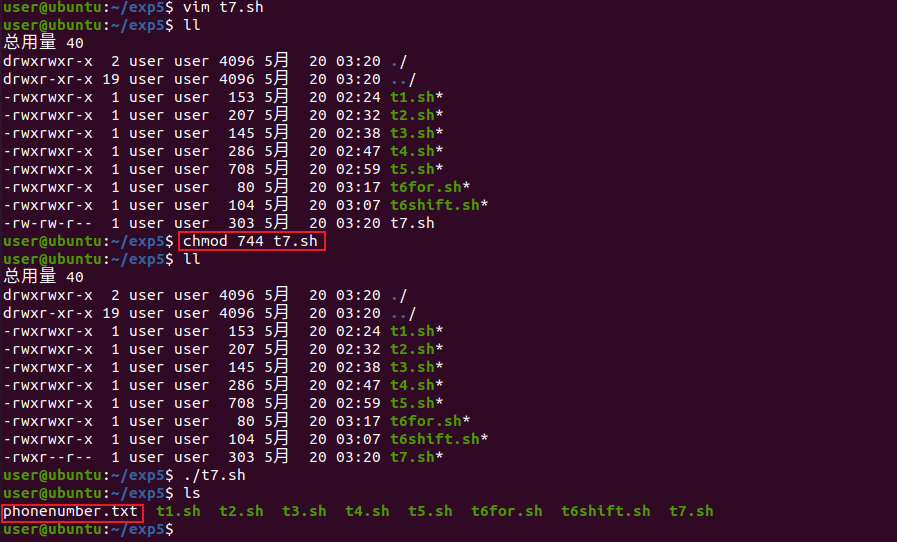8.在第7题产生的1000个电话号码中抽取5位幸运号码，并显示，要求只显示前3三位数字和后4位数字，中间四位用*代替。

#!/bin/bash
phone=./phonenumber.txt
for ((i=1;i<=5;i++))
do
line=wc -l $phone | cut -d' ' -f1 luck_line=$[RANDOM%$line+1] luck_number=head -$luck_line $phone | tail -1 echo "139****${luck_number:7:4}"
echo $luck_number >> luck.txt sed -i "/$luck_number/d" $phone done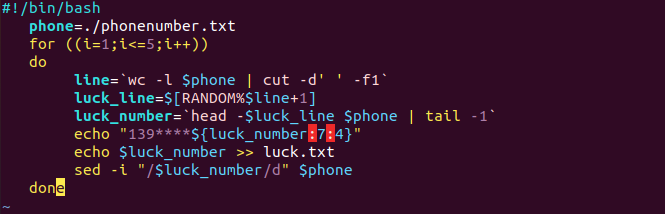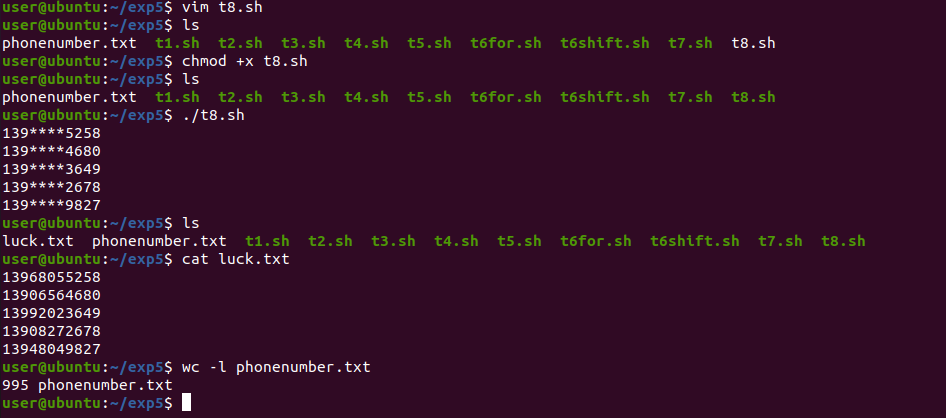9.使用until语句批量创建10个用户，要求stu1-stu5用户的UID分别为2001-2005，stu6-stu10用户的家目录分别在/rhome/stu6-/rhome/stu10。 #!/bin/bash if [ -d /rhome ] then echo "/rhome目录已存在" else mkdir /rhome echo "/rhome不存在，已创建成功" fi i=1 until [$i -gt 5 ]
do
useradd stu$i -u$[2000+$i] && echo "stu$i:123" | chpasswd && echo "stu$i创建成功" let i++ done until [$i -gt 10 ]
do
useradd stu$i -d /rhome/stu$i && echo "stu$i:123" | chpasswd && echo "stu$i创建成功"
let i++
done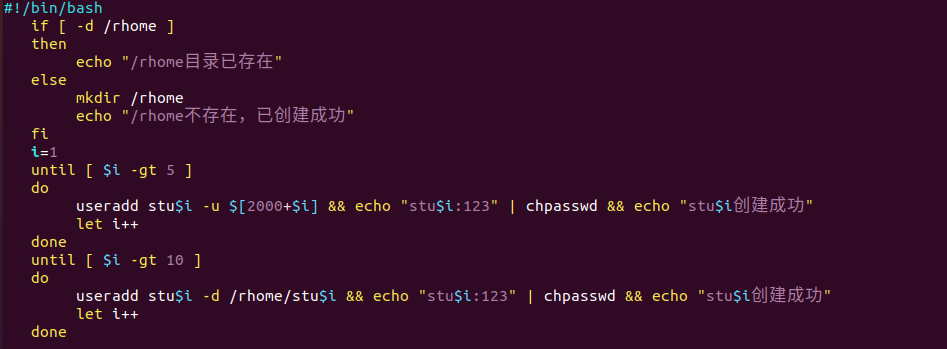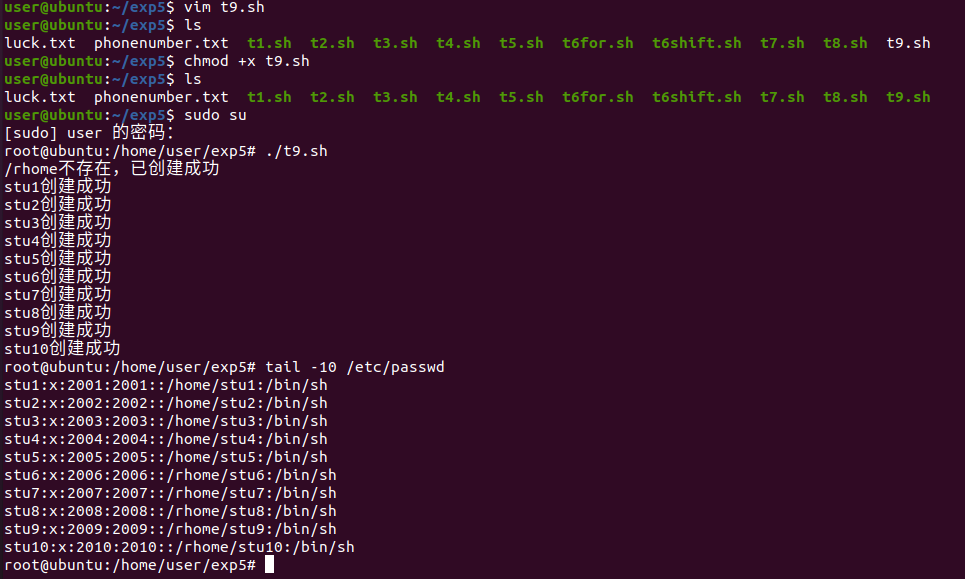10.编写脚本收集用户输入的基本信息（姓名，性别，年龄），如果用户不输入一直提示用户输入，最后根据用户输入的信息输出相应的内容。

#!/bin/bash
input_fun(){
input_var=""
output_var=$1 while [ -z$input_var ]
do
read -p "$output_var" input_var done echo$input_var
}

name=input_fun 请输入你的姓名:
sex=input_fun 请输入你的性别:
age=input_fun 请输入你的年龄:

echo "你的姓名是$name" echo "你的性别是$sex"
echo "你的年龄是\$age"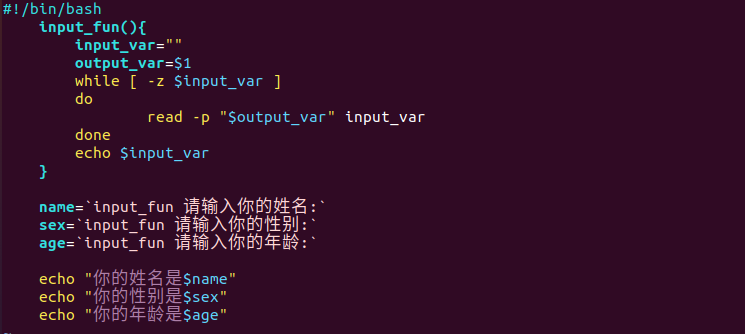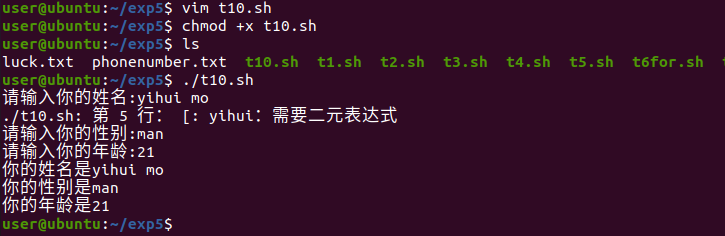posted @ 2021-05-20 18:49  codebuilder  阅读(102)  评论(0编辑  收藏  举报